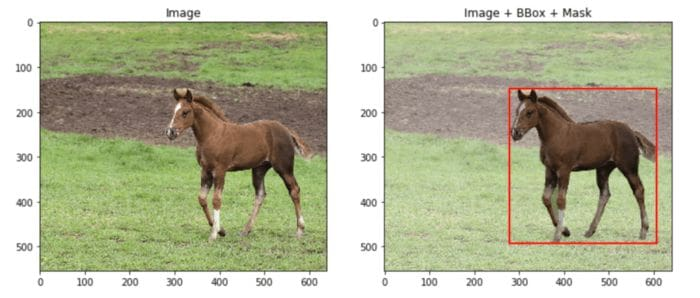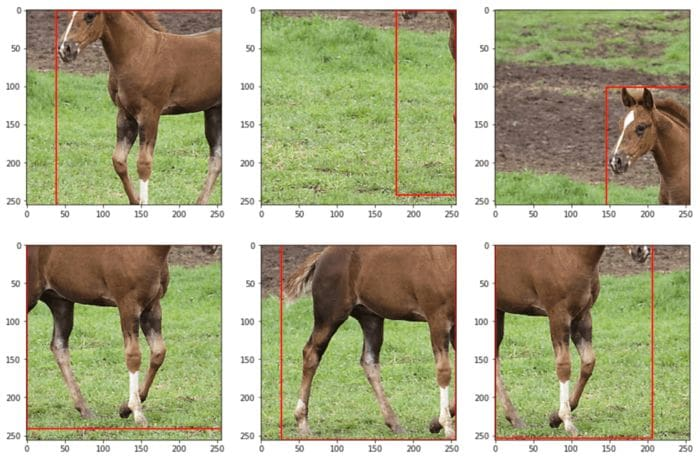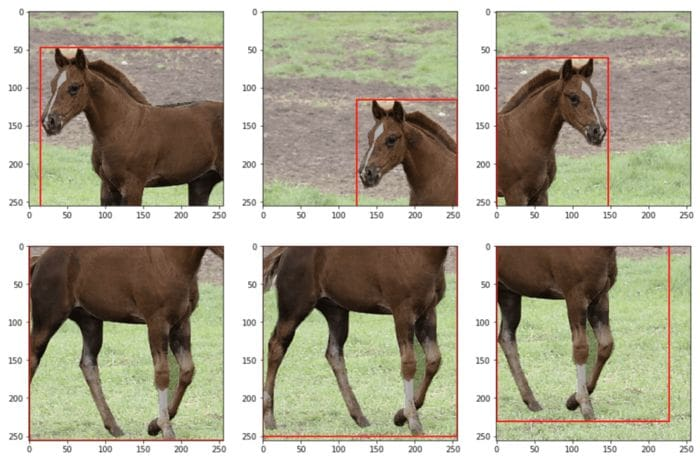By Olga Chernytska, Senior Machine Learning Engineer

Native PyTorch and TensorFlow augmenters have a big disadvantage – they cannot simultaneously augment an image and its segmentation mask, bounding box, or keypoint locations. So there are two options – either write functions on your own or use third-party libraries. I tried both, and the second option is just better 🙂

### Why Albumentations?

Albumentations was the first library that I’ve tried, and I’ve stuck with it, because:

• It is open-source,
• Intuitive,
• Fast,
• Has more than 60 different augmentations,
• Well-documented,
• And, what is most important, can simultaneously augment an image and its segmentation mask, bounding box, or keypoint locations.

There are two more similar libraries – imgaug and Augmentor. Unfortunately, I cannot provide any comparison, as I haven’t tried them yet. Till this moment Albumentations was just enough.

### Short Tutorial

In this short tutorial, I’ll show how to augment images for segmentation and object detection tasks – easily with few lines of code.

If you’d like to follow this tutorial:

1. Install Albumentations. I really recommend checking if you have the latest version, as older ones may be buggy. I use version ‘1.0.0’ and it works fine.
2. Download a test image with labels below. It is just a random image from COCO dataset. I modified it a bit and stored it in the format required by Albumentations. This library accepts images as NumPy arrays, segmentation masks as NumPy arrays, and bounding boxes as lists.

Let’s load the image, its binary pixel-wise segmentation mask, and a bounding box. The bounding box is defined as a 4-element list – [x_min, y_min, width, height].

``````import pickle
import numpy as np
import matplotlib.pyplot as plt
import matplotlib.patches as patches

with open("image_data.pickle", "rb") as handle:

image = image_data["image"]
bbox = image_data["bbox_coco"]

# visualize data
fig, ax = plt.subplots(1, 2, figsize=(12, 5))
ax.imshow(image)
ax.set_title("Image")
ax.imshow(image)
bbox_rect = patches.Rectangle(
bbox[:2], bbox, bbox, linewidth=2, edgecolor="r", facecolor="none"
)
plt.show()``````Image. The output when running code for image and its labels visualization.
Segmentation mask is visualized as a transparent black-white image (1 is black, ‘horse’).

Mask Augmentation for Segmentation. And now we can start with Albumentations. Transformations here are defined very similarly to PyTorch and TensorFlow (Keras API):

• Define transformation via combining several augmentations using a Compose object.
• Each augmentation has argument `p`, the probability to be applied, and additionally augmentations-specific arguments, like `width` and `height` for RandomCrop.
• Use defined transformation as a function to augment the image and its mask. This function returns a dictionary with keys – `image` and `mask`.

Below is the code on how to augment the image (and its mask) with random 256×256 crop (always) and horizontal flip (only in 50% cases).

``````import albumentations as A

# define augmentation
transform = A.Compose([
A.RandomCrop(width=256, height=256, p=1),
A.HorizontalFlip(p=0.5),
])

# augment and visualize images
fig, ax = plt.subplots(2, 3, figsize=(15, 10))
for i in range(6):
ax[i // 3, i % 3].imshow(transformed["image"])
ax[i // 3, i % 3].imshow(transformed["mask"], alpha=0.3, cmap="gray_r")
plt.show()``````

As a result, you should get something like this. Your augmented images will be different, as Albumentations produces random transformations. For a detailed tutorial on mask augmentation refer to original documentation.Image. The output when running code for simultaneous image and mask augmentation.
Segmentation mask is visualized as a transparent black-white image (1 is black, ‘horse’)

Bounding Boxes Augmentation for Object Detection. It is similar to augmentation for segmentation masks, however:

• Additionally, define `bbox_params`, where specify the format of the bounding box and argument for bounding box classes. `coco` means bounding box in COCO dataset format – [x_min, y_min, width, height]. And argument `bbox_classes` will be used later to pass classes for bounding boxes.
• `transform` accepts bounding boxes as a list of lists. Additionally, it requires bounding box classes (as a list) even if there is a single bounding box in the image.

Below is the code that does RandomCrop and HorizonalFrip simultaneously for the image and its bounding box.

``````# define augmentation
transform = A.Compose([
A.RandomCrop(width=256, height=256, p=1),
A.HorizontalFlip(p=0.5),
], bbox_params=A.BboxParams(format="coco", label_fields=["bbox_classes"]))

# augment and visualize images
bboxes = [bbox] #`transform` accepts bounding boxes as a list of lists.
bbox_classes = ["horse"]

fig, ax = plt.subplots(2, 3, figsize=(15, 10))
for i in range(6):
transformed = transform(
image=image,
bboxes=bboxes,
bbox_classes=bbox_classes
)
ax[i // 3, i % 3].imshow(transformed["image"])
trans_bbox = transformed["bboxes"]
bbox_rect = patches.Rectangle(
trans_bbox[:2],
trans_bbox,
trans_bbox,
linewidth=2,
edgecolor="r",
facecolor="none",
)
ax[i // 3, i % 3].add_patch(bbox_rect)
plt.show()``````

And here are the results. In case you need some specific bounding box augmentations – refer to the original documentation.Image. The output when running code for simultaneous image and bounding box augmentation.

Simultaneous augmentation of multiple targets. Besides allowing to simultaneously augment several masks or several bounding boxes, Albumentations has a feature to simultaneously augment different types of labels, for instance, a mask and a bounding box.

When calling a `transform` simply give it everything you have:

``````# define augmentation
transform = A.Compose([
A.RandomCrop(width=256, height=256, p=1),
A.HorizontalFlip(p=0.5),
], bbox_params=A.BboxParams(format="coco", label_fields=["bbox_classes"]))

# augment and visualize images
bboxes = [bbox]
bbox_classes = ["horse"]

fig, ax = plt.subplots(2, 3, figsize=(15, 10))
for i in range(6):
transformed = transform(
image=image,
bboxes=bboxes,
bbox_classes=bbox_classes
)
ax[i // 3, i % 3].imshow(transformed["image"])
trans_bbox = transformed["bboxes"]
bbox_rect = patches.Rectangle(
trans_bbox[:2],
trans_bbox,
trans_bbox,
linewidth=2,
edgecolor="r",
facecolor="none",
)
ax[i // 3, i % 3].add_patch(bbox_rect)
ax[i // 3, i % 3].imshow(transformed["mask"], alpha=0.3, cmap="gray_r")
plt.show()``````

Your result will look like in the image below. And here is more detailed documentation on that.Image. The output when running code for a simultaneous image, segmentation mask, and bounding box augmentation.
Segmentation mask is visualized as a transparent black-white image (1 is black, ‘horse’).

And More. Albumentations has much more features available, such as augmentation for keypoints and AutoAugment. And it includes about 60 different augmentation types – literally for any task you need.

### Compatibility with PyTorch & TensorFlow

Most likely you are going to use Albumentations as a part of PyTorch or TensorFlow training pipeline, so I’ll briefly describe how to do it.

PyTorch. When creating a Custom dataset, define Albumentations transform in the `__init__` function and call it in the `__getitem__` function. PyTorch models require input data to be tensors, so make sure you add `ToTensorV2` as the last step when defining `transform` (a trick from one of Albumentations tutorials).

``````from torch.utils.data import Dataset
from albumentations.pytorch import ToTensorV2

class CustomDataset(Dataset):
self.images = images  # assume it's a list of numpy images
self.transform = A.Compose([
A.RandomCrop(width=256, height=256, p=1),
A.HorizontalFlip(p=0.5),
ToTensorV2(),
])

def __len__(self):
return len(self.images)

def __getitem__(self, idx):
image = self.images[idx]
transformed_image = transformed["image"]

TensorFlow (Keras API) also allows creating Custom datasets, similar to PyTorch. So define Albumentations transform in the `__init__` function and call it in the `__getitem__` function. Pretty simple, isn’t it?

``````from tensorflow import keras

class CustomDataset(keras.utils.Sequence):
self.images = images
self.batch_size = 1
self.img_size = (256, 256)
self.transform = A.Compose([
A.RandomCrop(width=256, height=256, p=1),
A.HorizontalFlip(p=0.5),
])

def __len__(self):
return len(self.images) // self.batch_size

def __getitem__(self, idx):
"""Returns a batch of samples"""
i = idx * self.batch_size
batch_images = self.images[i : i + self.batch_size]
batch_images_stacked = np.zeros(
(self.batch_size,) + self.img_size + (3,), dtype="uint8"
)
(self.batch_size,) + self.img_size, dtype="float32"
)
for i in range(len(batch_images)):
transformed = self.transform(
image=batch_images[i],